# John's Online RPN Scientific Calculator, using Complex Math

A powerful, online, RPN Scientific Calculator, written in Javascript. It employs complex math, has hundreds of functions (including complex Gaussian integer factorization, complex roots of unity, combinatorics, the 12-fold way, continued fraction approximations, etc.), and a scrollable display/log window. This is an example of just how powerful the javascript language can be. The true test of a real calculator: Sqrt[-1]. If you get an imaginary answer, then you have a TRUE calculator. If it crashes, then toss it and go get a TRUE calculator... You can also write Advanced DAL (Dynamic/Direct Algebraic Logic) equations directly to the input window as well. There is a memory slot with an unlimited number of entries and the standard Store/Recall/Clear function. Since you can cut/copy/paste and select/drag from the scrollable log window, you can even use the log window as an effective memory. It has buttons for various constants, complete trigonometric functions, integer and modular math functions such as integer factorization and gcd, base conversion from 2 to 36, not just bin, oct, dec, & hex, etc. Input numbers from the mouse-click pad or from the keyboard. Also includes an NxN linear system solver and N-polynomial complex root finder which can have complex coefficients.
See also, my Online SR 4-Vector & Tensor Mathematics Calculator SR 4-Vector & Tensor Mathematics CalculatorSiteMap of SciRealm | About John | John's Resume/C.V./Bio | Send email to John John's Science, Math, Philosophy Stuff: The Science Realm: John's Virtual Sci-Tech Universe | 4-Vectors | Ambigrams | Antipodes | Covert Ops Fragments | Cyrillic Projector | Forced Induction (Sums Of Powers Of Integers) | Fractal Tree | Frontiers | IFS Fractals (Assembly) | IFS Fractals (Javascript) | JavaScript Graphics | Kid Science | Kryptos | Philosophy | Photography | Physics-Planck Constant Measurement Via LEDs | Prime Sieve | QM from SR | QM from SR-Simple RoadMap | QM from SR-Simple RoadMap (PDF) | SR 4-Vector & Tensor Calculator |Quantum Phase | Quotes | RuneQuest Cipher Challenge | Secret Codes & Ciphers | Scientific Calculator (complex capable) | Science+Math | Sci-Pan Pantheist Poems | Stereograms | SuperMagicSqr4x4 |Turkish Grammar |Melike Wilson Art Site last modified: 2022 Welcome to Relativistic Quantum Reality: Virtual worlds of imaginary particles: The dreams stuff is made of: Life, the eternal ghost in the machine... This site is dedicated to the quest for knowledge and wisdom, through science, mathematics, philosophy, invention, and technology.  May the treasures buried herein spark the fires of imagination like the twinkling jewels of celestial light crowning the midnight sky... Quantum Mechanics is derivable from Special Relativity See SRQM - QM from SR - Simple RoadMap (.html) See SRQM - QM from SR - Simple RoadMap (.pdf) See SRQM - QM from SR - Summary (.pdf) See SRQM - Online SR 4-Vector & Tensor Calculator See Online Complex-capable RPN Scientific Calculator ***Learn, Discover, Explore***Rect Polar ExpPh.D. SystemsRPN ScientificCalculator re or (re,im) list {z1;z2;z3} KBD rad deg grad cycl hyp inv > FunctionList Tips Help Tips Fncs Help Fncs

Logic Calculator:

xxx

Complex Linear System Equation Solver: - you can use complex coefficients in form of (re,im)

xxx

Polynomial Root Solver: Lin-Bairstow method

xxx

Complex Polynomial Root Solver: - you can use complex coefficients in form of (re,im)
uses Modified Durand-Kerner-Weierstrass Method (iterated)
if you are expecting integer results, try hitting the solve button several times to reset the initial guesses

xxx

Javascript now has the ability to generate respectable graphics
John's Online Complex-capable RPN SciCalculator
Math-CalculusDerivativeRulesChart.pdf
Math-GraphingEquations.html
Math-L-SystemFractals.html
Math-LogisticMapChaos.html
Math-MeasuringSpaceFractal.html
Math-PlasmaFractal.html
Math-RhodoneaCurve.html
Math-Spirograph.html
Stereograms : 3D Rotating Light Array in JavaScript : 3D Intersection Demo in JavaScript : IFS Fractals in JavaScript : TreeFractal : Physics-PlanckConstantViaAtomicLineSpectra.html
Physics-PlanckConstantViaComptonScattering.html
Physics-PlanckConstantViaElectronDiffraction.html
Physics-PlanckConstantViaGravityInducedInterferometry
Physics-PlanckConstantViaIncandescence.html
Physics-PlanckConstantViaLEDs.html
Physics-PlanckConstantViaSagnacEffect.html
Physics-PlanckConstantViaSternGerlach.html
or try functions below

 To use Scientific Calculator RPN (Reverse Polish Notation) button operators (Complex operation capable): For unary operations (1 number req.), type the number into the input box and then click the function. For binary operations (2 numbers req.) type the 1st number, click the [Enter] button, type the 2nd number, and then click the function. For short-cut RPN operations (like HP calculator logic), you can use operators directly on the stack as well. Unary operations replace the last stack value with its new value Binary operations replace the last two stack values with its new value Just play with the buttons, the log window will let you see how it works -- its pretty easy once you get the hang of it... To use built-in javascript DAL (Dynamic/Direct Algebraic Logic) operations (Real operations only): Write your numeric equation/formula directly into the input box and then press [Enter] (you don't have to write the JS 'Math.' in front of the functions, but you can only use and receive real numbers): ex. 2+5/3-abs(-8)*cos(PI) you must input angles in radians when using this mode Note: you must have the [KBD] checkbox OFF to use DAL To input numbers, there are several options, all of which are effective: (1) mouse-click on the virtual number pad above (2) use copy-paste/select-drag from the log window to the input box (3) just type in the numbers on your computer keyboard (4) turn on the [KBD] checkbox to make the actual keyboard activate the (+,-,*,/,=,c) virtual keys in RPN mode Input types: real 255 or PI or -234.2235 or etc. complex pair (0,3) or (2,3) or (-2,3) etc. complex add 0 +i 3 or 2 +i 3 or -2 +i 3 etc. imaginary 3i or 2+3i or -2+3i or 2+3I etc. complex polar 3 /_ 90  or   3 /_ 1.5708 (set the angle mode 1st) num-to-base   FF_b16 or 1001010_b2 list of complex {2;(3,4.5);4.46;(5,3);3;4;(5.23,-4.84);2} Javascript numbers: octal integer �0### 0345 -> 229 decimal  -0345 -> -229 decimal hexadecimal integer �0x### 0x345 -> 837 decimal -0x345 -> -837 decimal To page through the available functions: Keep clicking the [Fncs] key Warning: Apparently, javascript only uses about 16 digits of precision, so take results of huge numbers with a grain of salt...

 To use Javascript Math functions in input field: === To use Javascript operators in input field (listed in precedence order): Math.abs(x) Absolute value of x ( , ) Parentheses for grouping Math.acos(x) Arc cosine of x ~ Bitwise complement Math.asin(x) Arc sine of x ! Logical complement Math.atan(x) Arc tangent of x typeof Return data type Math.atan2(y,x) Argument from +x axis * , / , % Multiply , Divide , Remainder Math.ceil(x) Ceiling of x + , - Add , Subtract Math.cos(x) Cosine of x + Concatenate strings Math.exp(x) Natural exponent of x << Integer shift left Math.floor(x) Floor of x >> Integer shift right, sign extension Math.log(x) Natural logarithm of x >>> Integer shift right, zero extension Math.max(args...) Max of list of values < , <= Less than , Less than or equal Math.min(args...) Min of list of values > , >= Greater than, Greater than or equal Math.pow(x,y) x to the y power == Test for equality Math.random() Random number (0..1) != Test for inequaltiy Math.round(x) Round of x === Test for identity Math.sin(x) Sine of x !== Test for identity Math.sqrt(x) Square root of x & Integer bitwise AND Math.tan(x) Tangent of x ^ Integer bitwise XOR | Integer bitwise OR Math.E Natural exponent E && Logical AND Math.LN10 Natural logarithm of 10 || Logical OR Math.LN2 Natural logarithm of 2 ?: Conditional operator "condition"?"if true":"if false" Math.LOG10E Base-10 log of E Math.LOG2E Base-2 log of E NaN Not a Number Math.PI Circle constant PI Math.SQRT1_2 Square root of 1/2 Math.SQRT2 Square root of 2
Note: all built-in javascript trig functions use radians in the argument
Note: if anyone notices a bug in the calculator, please report it so that I can fix it

And, just an interesting bit of math trivia:

Addition is repeated incrementing ( c = a+b ); the 2 inverses are subtraction. ( a = c-b ) & ( b = c-a )
Multiplication is repeated addition ( c = a*b ); the 2 inverses are division. ( a = c/b ) & ( b = c/a )
Exponentiation is repeated multiplication ( c = a^b); it has 2 different inverses: the root ( a = Rootb[c] ) and the log ( b = Loga[c])
Isn't this branching pretty cool, considering that mathematics has a tree-like structure? :-)

Contest: Who can tell me what is special about 0.20787957635076193?
Hint: it can be derived using a single simple "unit" number and one single operation from above.
Video Introduction to John''s Scientific Calculator
th Email me, especially if you notice errors (which I will fix ASAP) or have interesting comments.## quantum relativity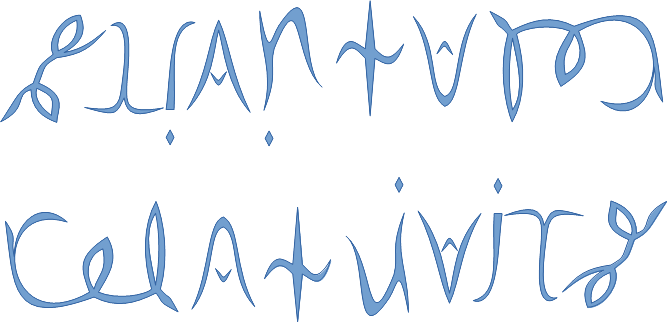See SRQM: QM from SR - The 4-Vector RoadMap (.html)
See SRQM: QM from SR - The 4-Vector RoadMap (.pdf)

SRQM Physics Diagramming Method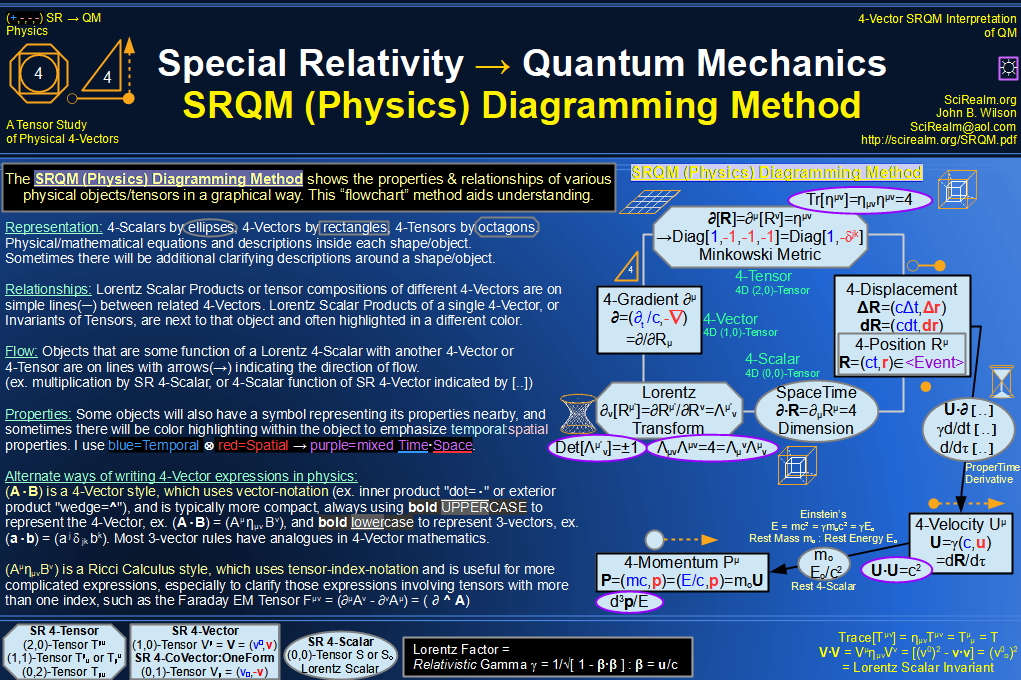SRQM 4-Vector : Four-Vector and Lorentz Scalar Diagram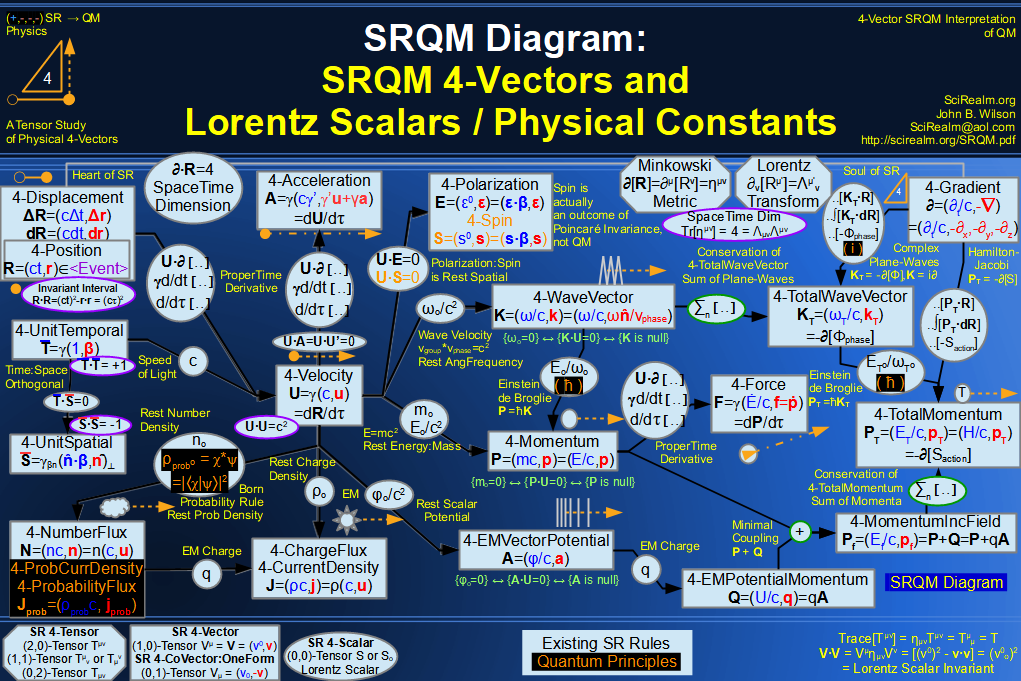SRQM + EM 4-Vector : Four-Vector and Lorentz Scalar Diagram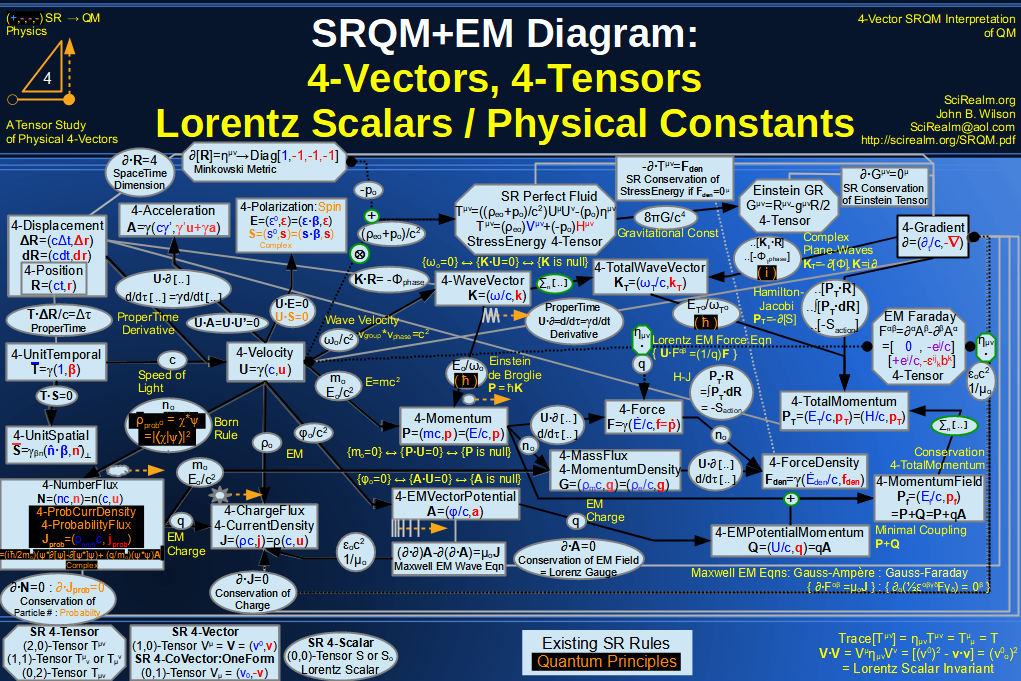SRQM + EM 4-Vector : Four-Vector and Lorentz Scalar Diagram With Tensor Invariants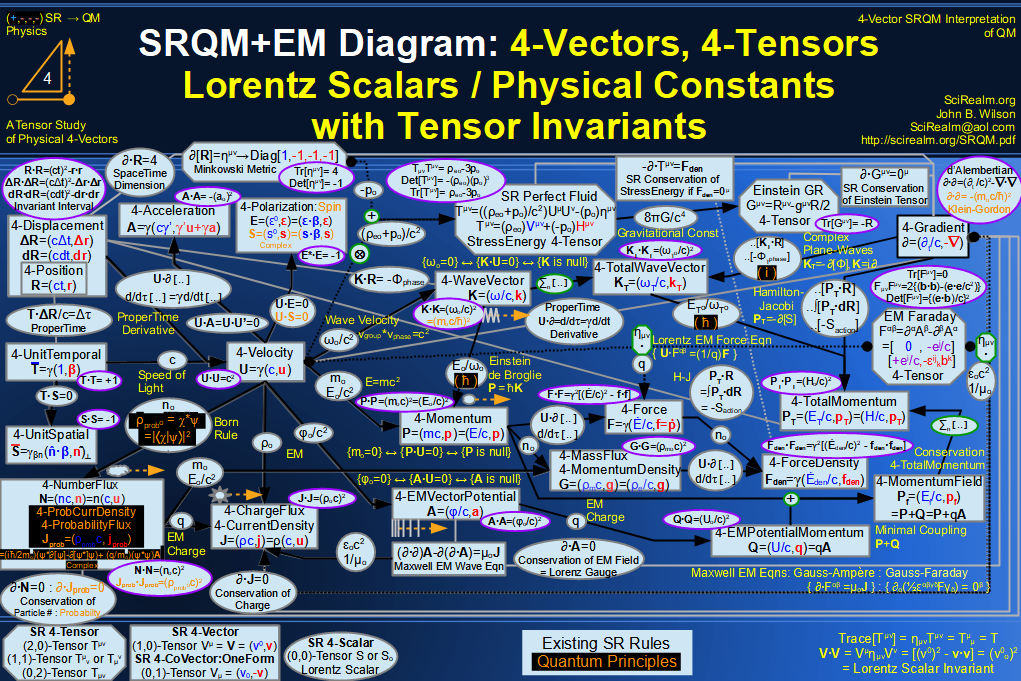SRQM 4-Vector : Four-Vector Stress-Energy & Projection Tensors Diagram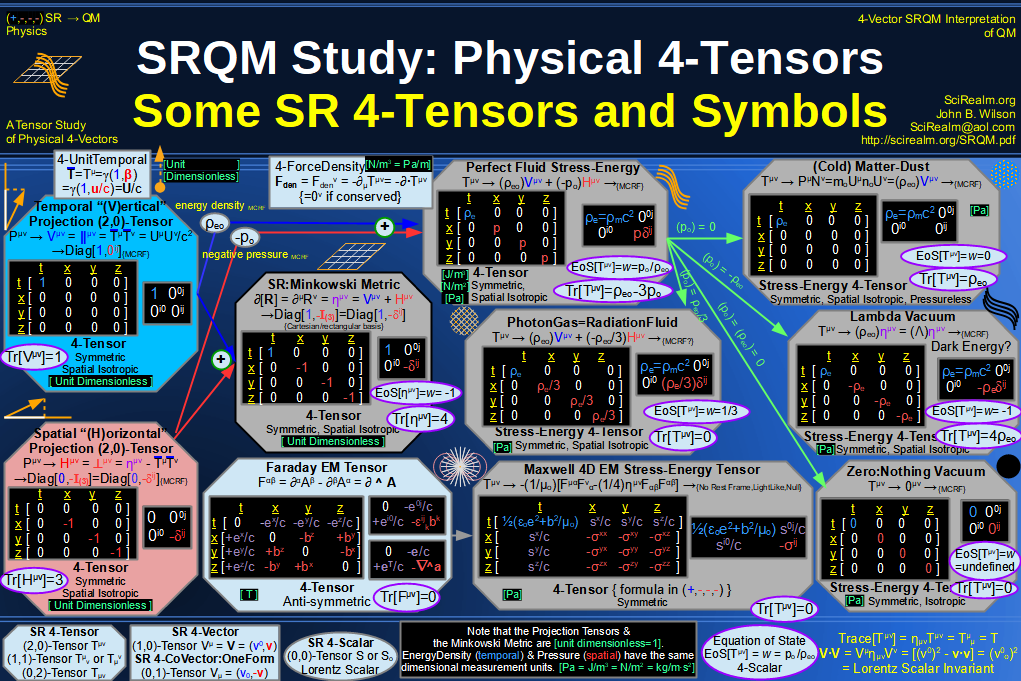SRQM 4-Vector : Four-Vector SR Quantum RoadMap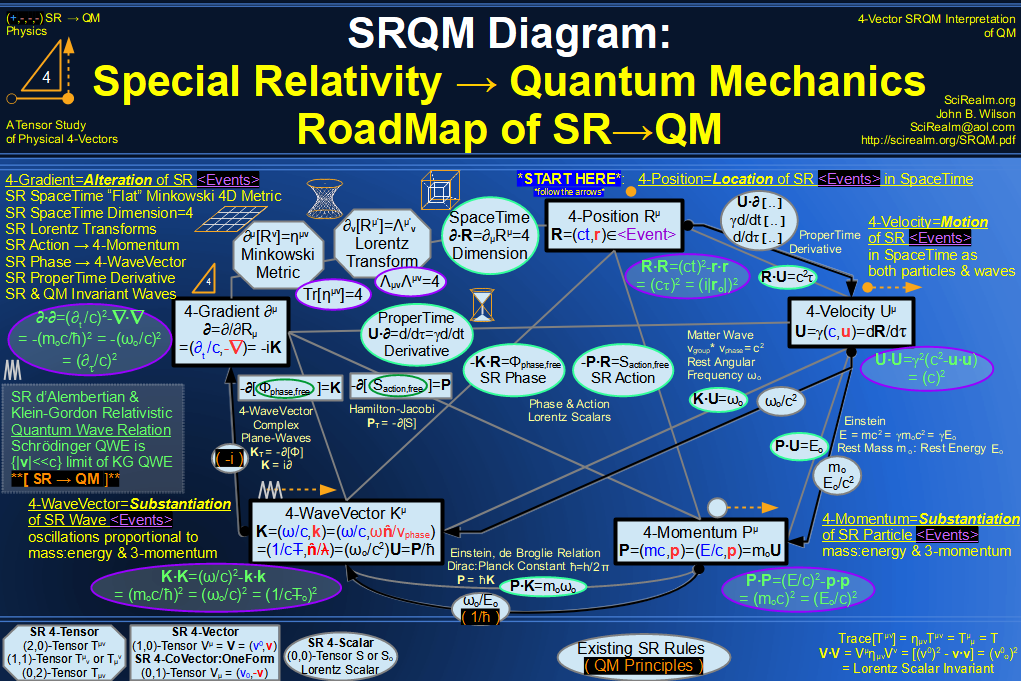SRQM + EM 4-Vector : Four-Vector SR Quantum RoadMap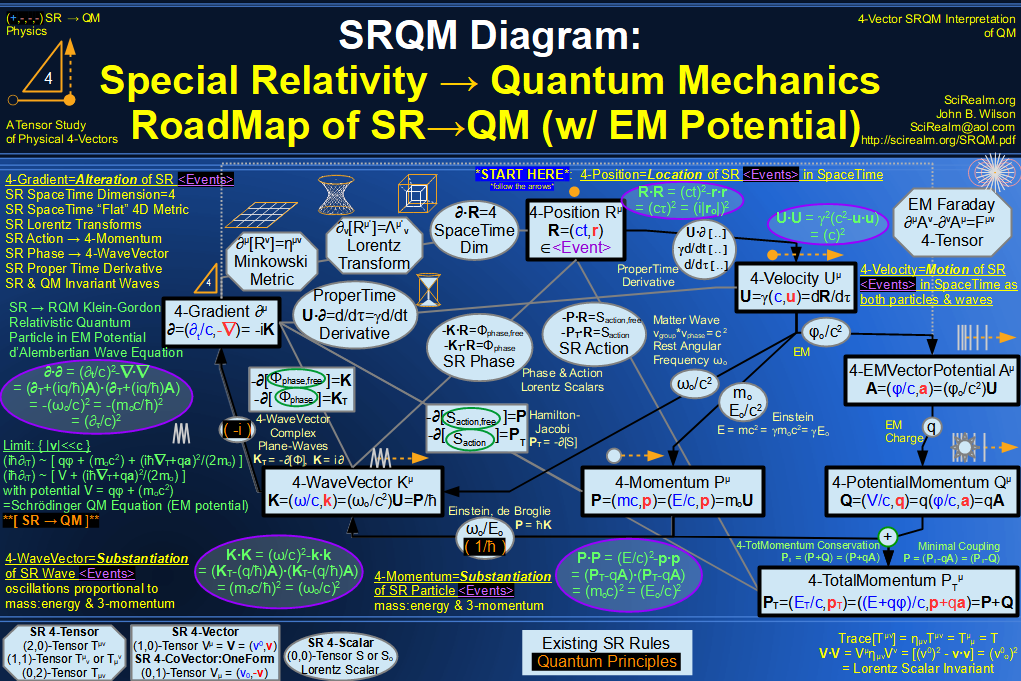SRQM + EM 4-Vector : Four-Vector SR Quantum RoadMap - Simple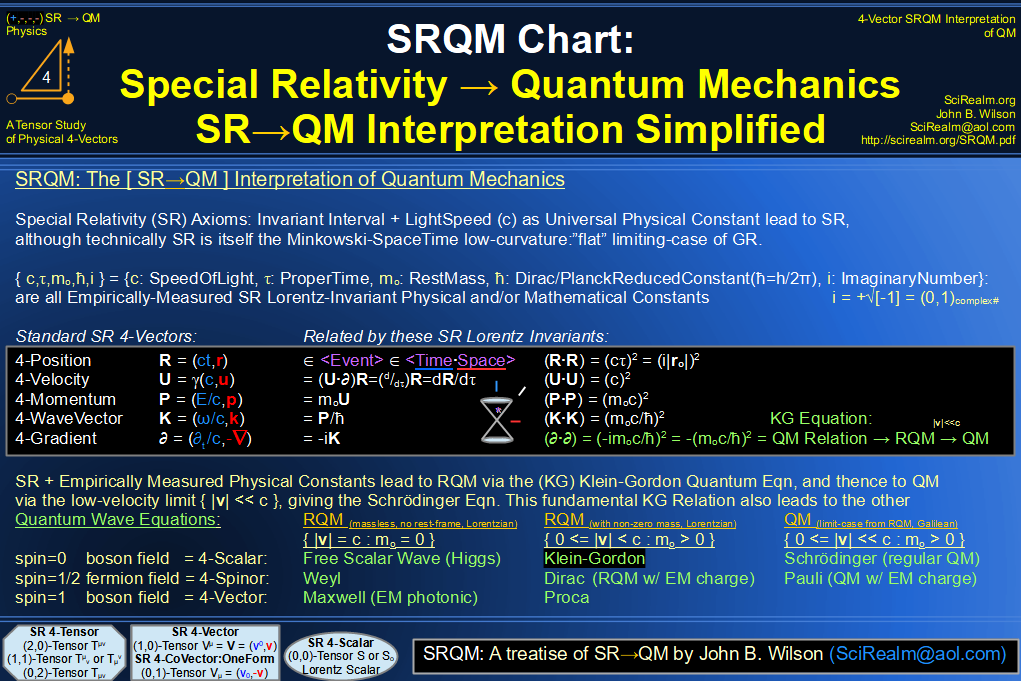SRQM 4-Vector : Four-Vector New Relativistic Quantum Paradigm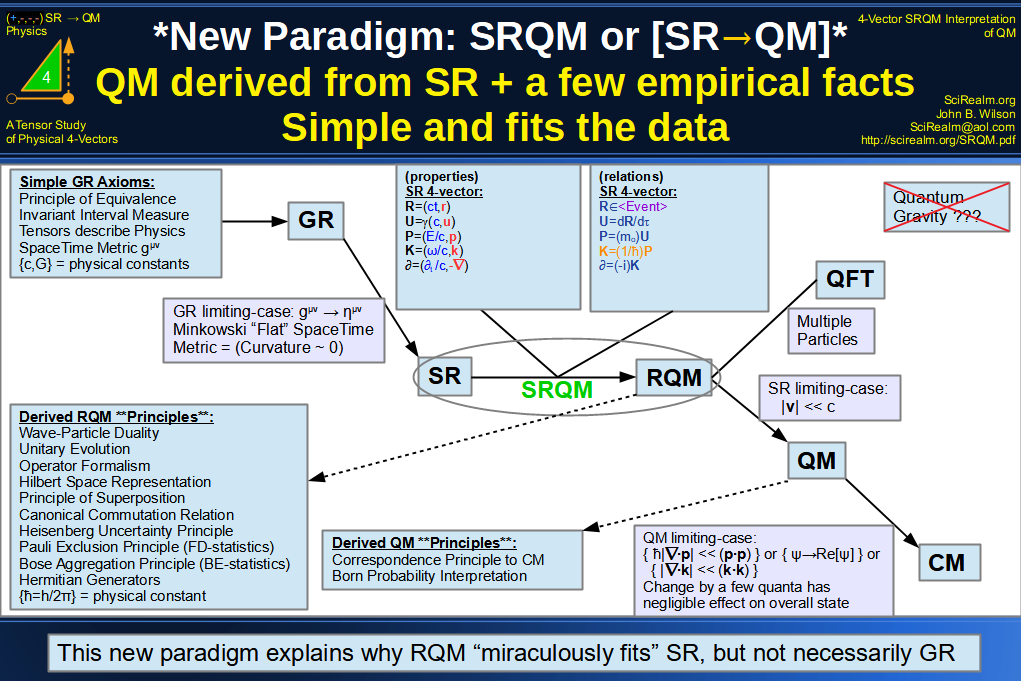SRQM + EM 4-Vector : Four-Vector New Relativistic Quantum Paradigm (with EM)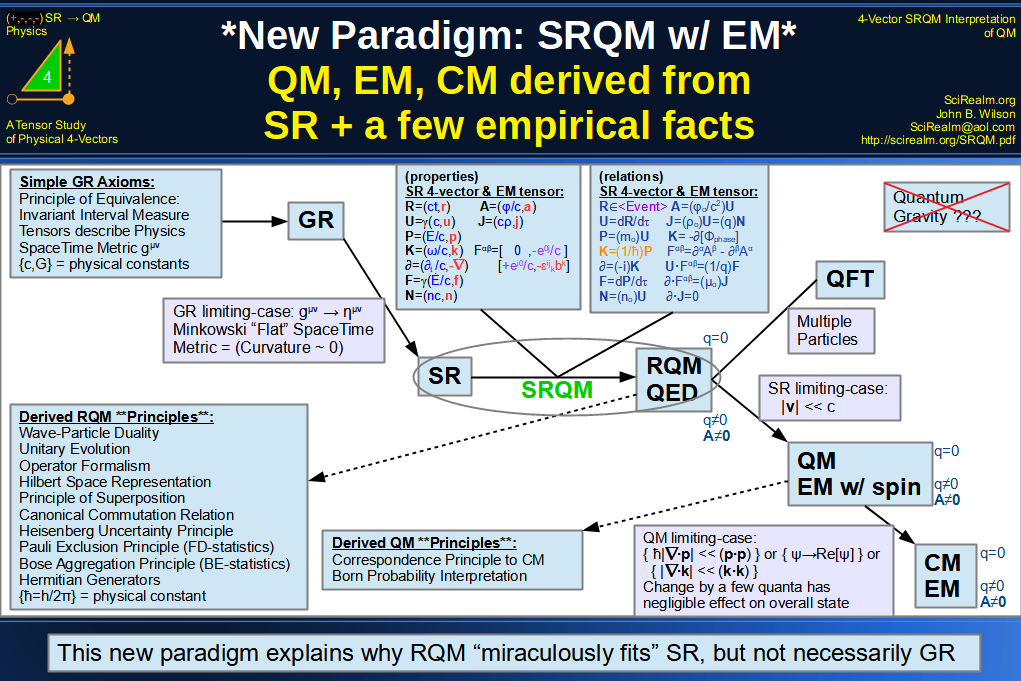SRQM 4-Vector : Four-Vector New Relativistic Quantum Paradigm - Venn Diagram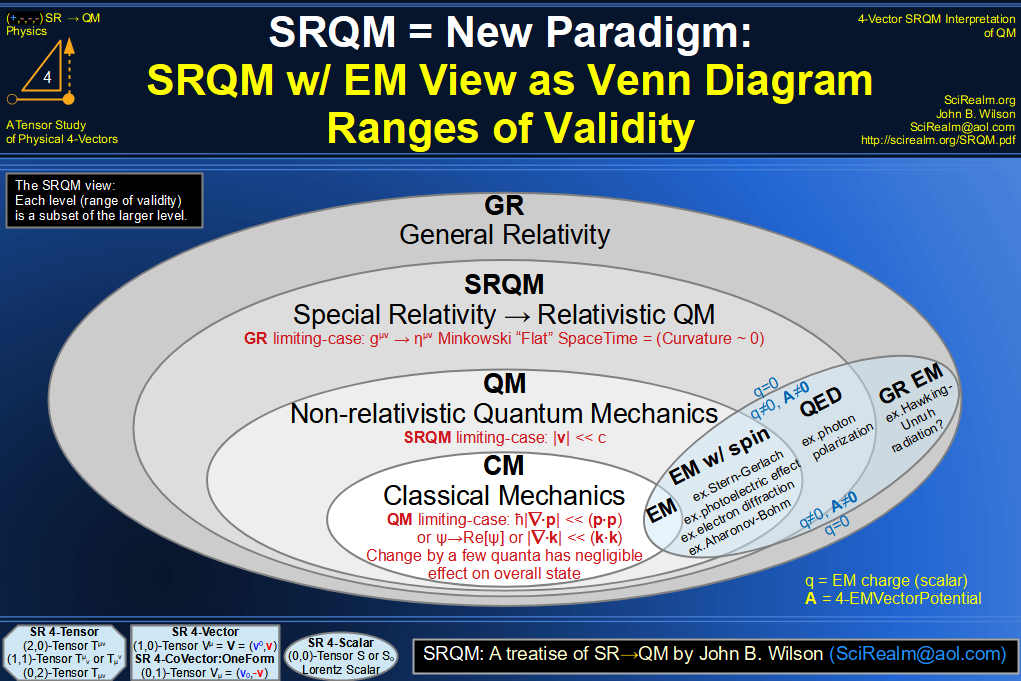SRQM 4-Vector : Four-Vector SpaceTime is 4D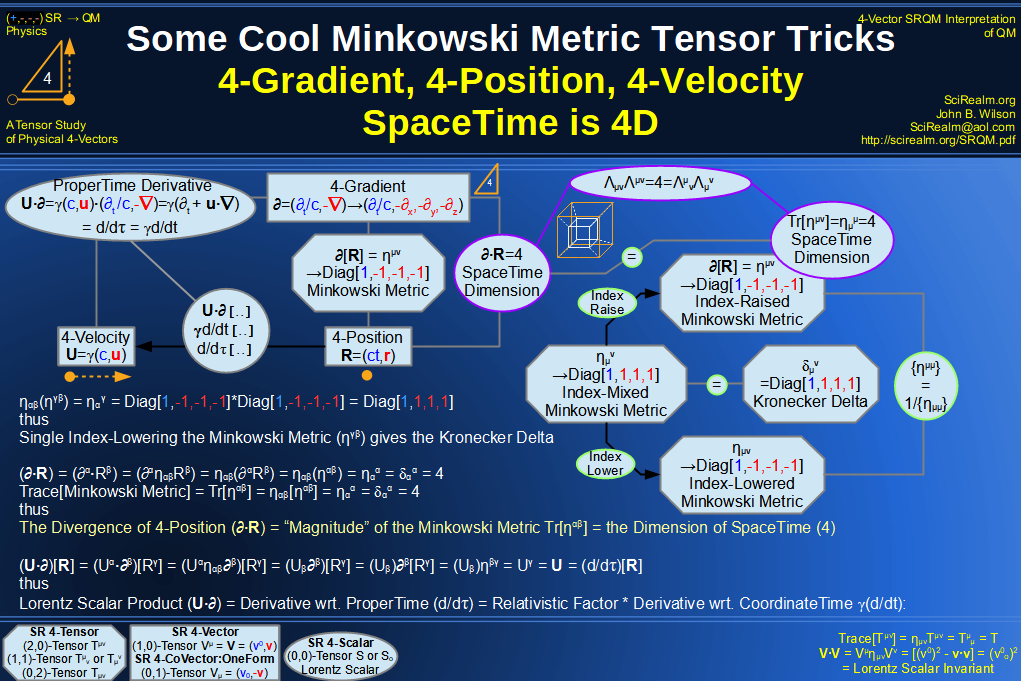SRQM 4-Vector : Four-Vector SpaceTime Orthogonality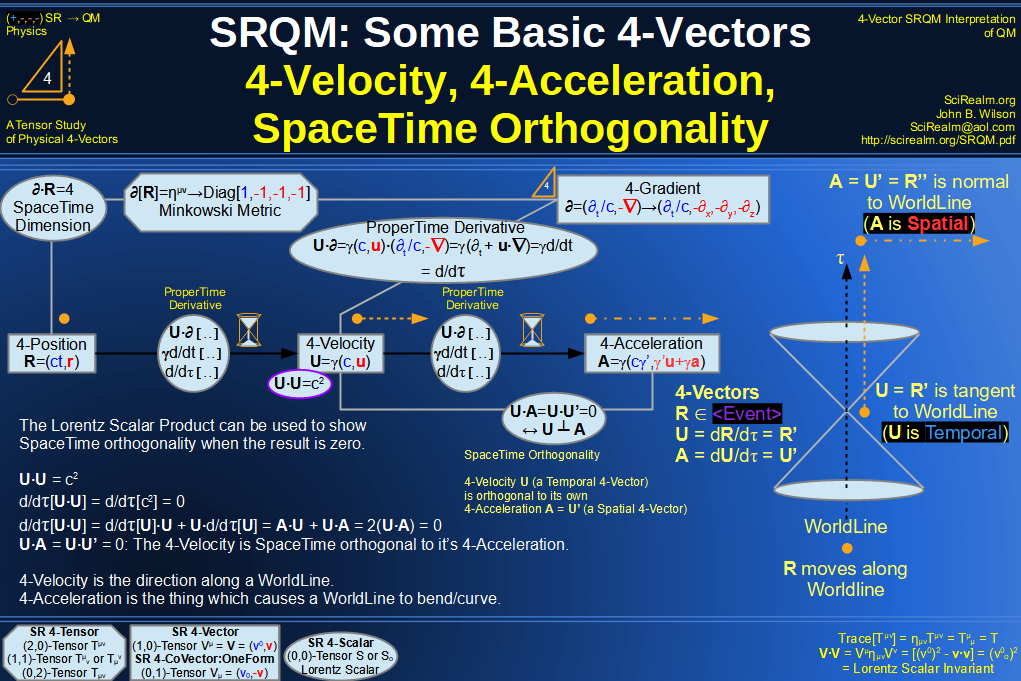SRQM 4-Vector : Four-Vector 4-Position, 4-Velocity, 4-Acceleration Diagram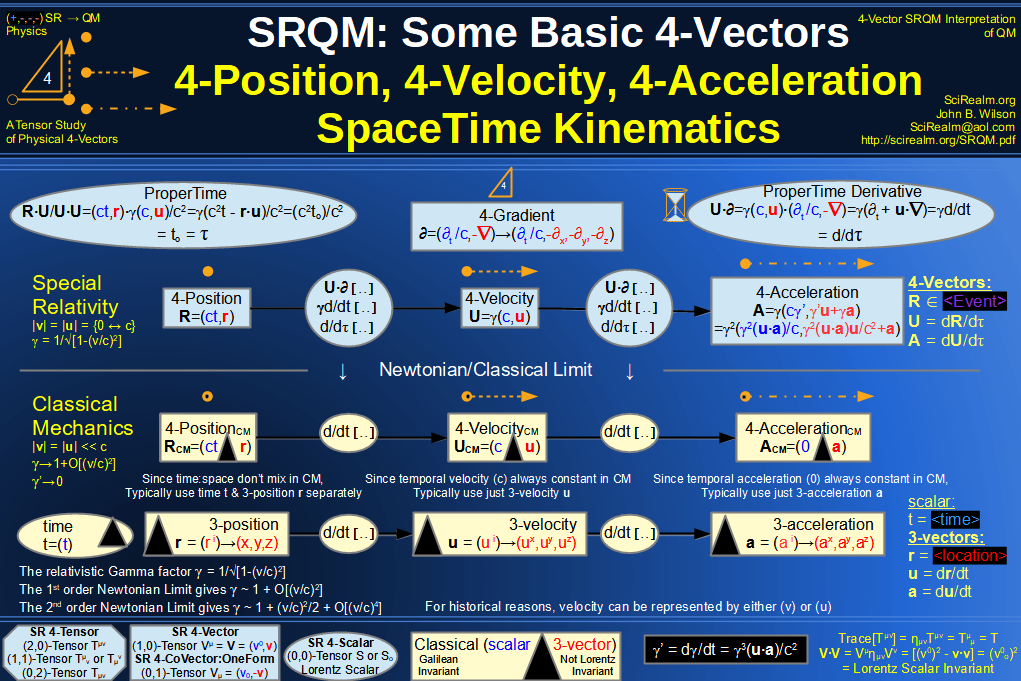SRQM 4-Vector : Four-Vector 4-Displacement, 4-Velocity, Relativity of Simultaneity Diagram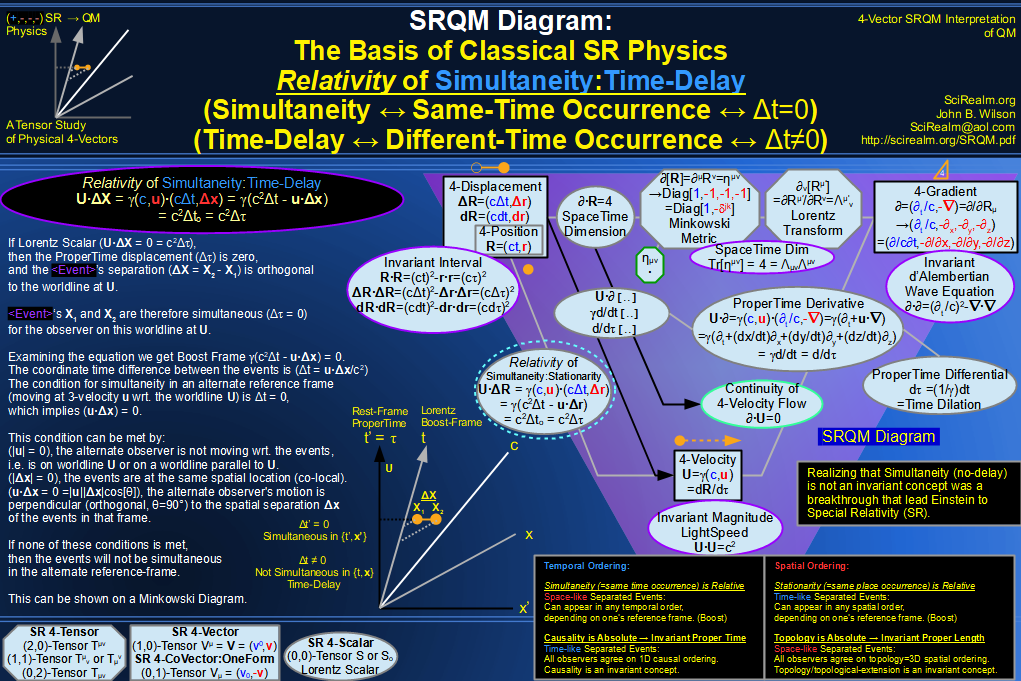SRQM 4-Vector : Four-Vector 4-Velocity, 4-Gradient, Time Dilation Diagram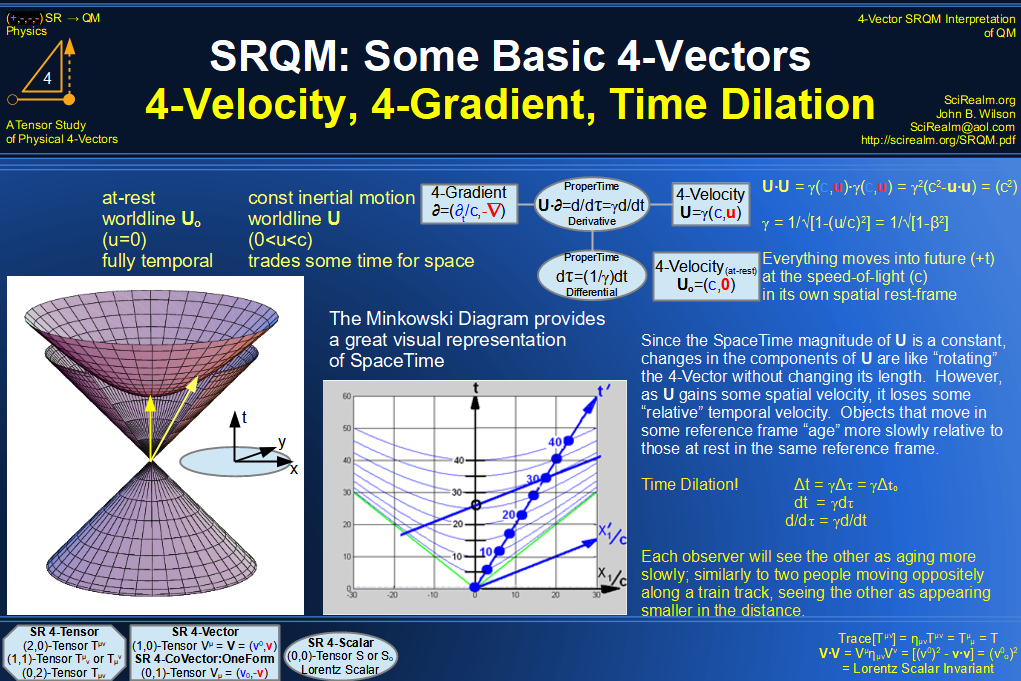SRQM 4-Vector : Four-Vector 4-Vector, 4-Velocity, 4-Momentum, E=mc^2 Diagram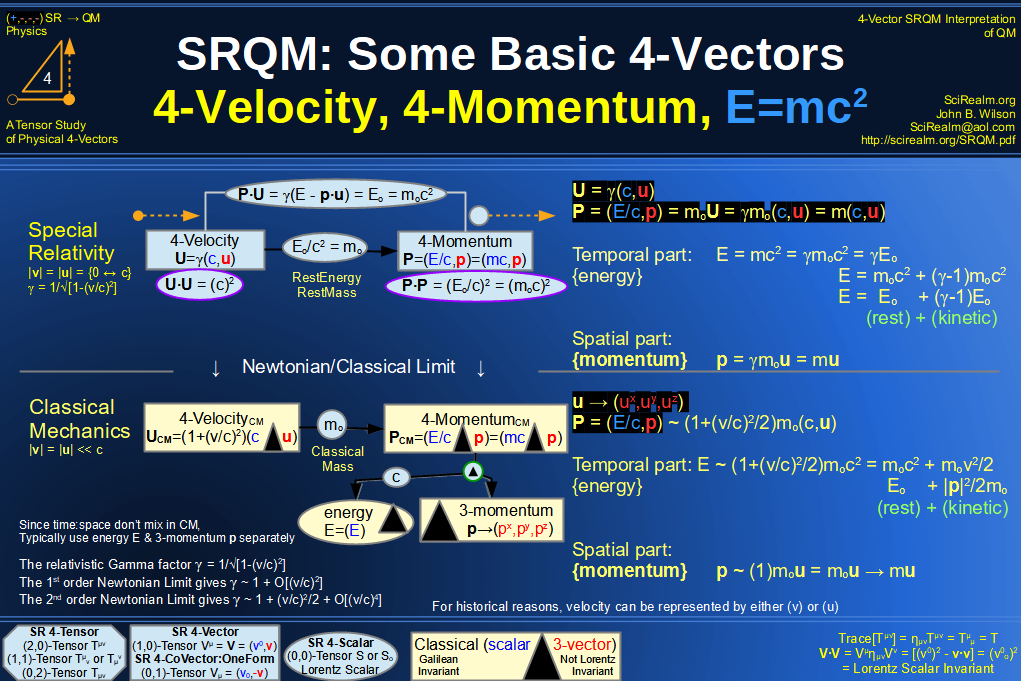SRQM 4-Vector : Four-Vector 4-Velocity, 4-WaveVector, Relativistic Doppler Effect Diagram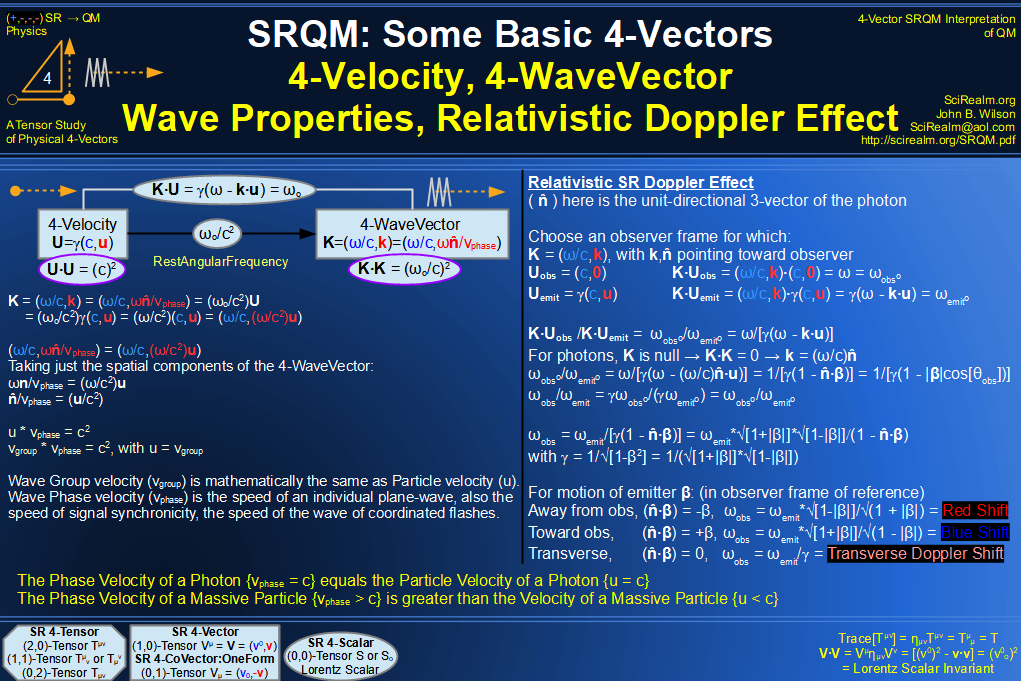SRQM 4-Vector : Four-Vector Wave-Particle Diagram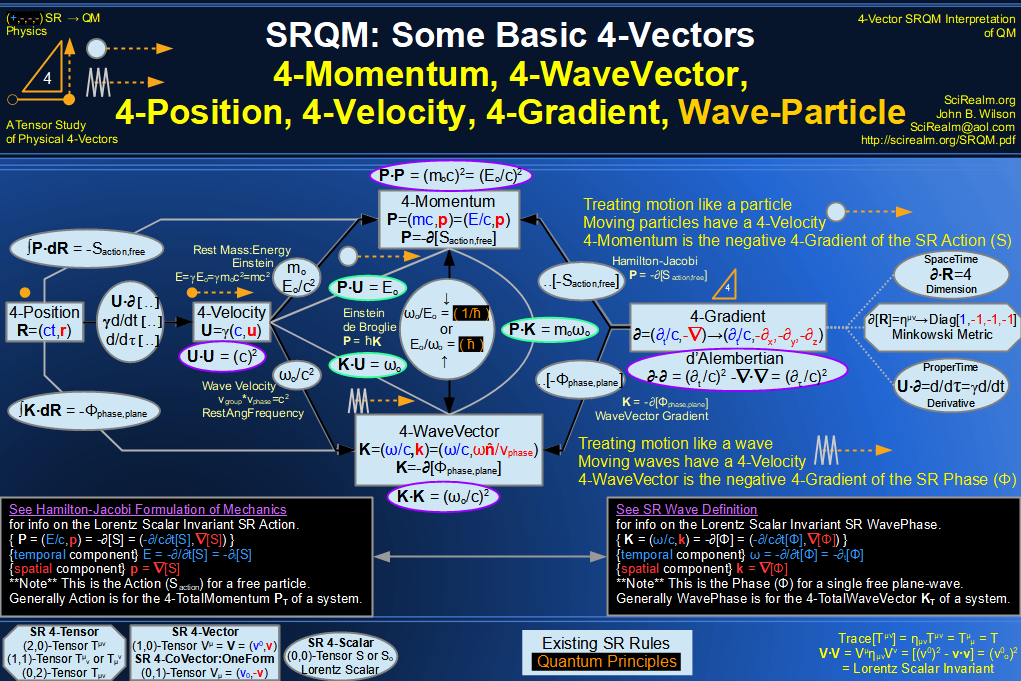SRQM 4-Vector : Four-Vector Compton Effect Diagram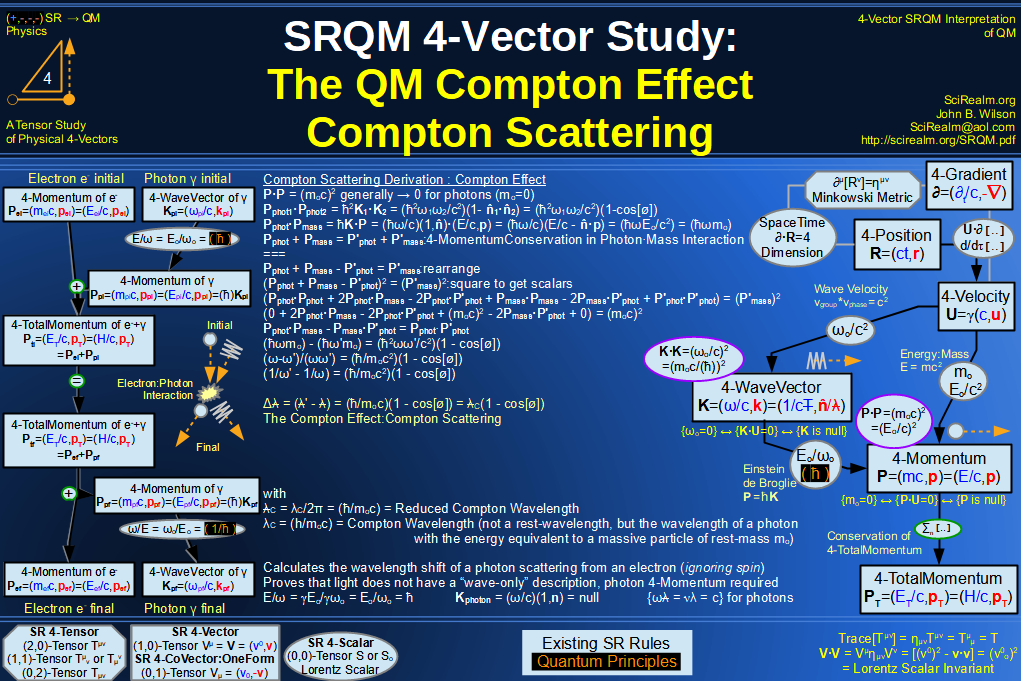SRQM 4-Vector : Four-Vector Aharonov-Bohm Effect Diagram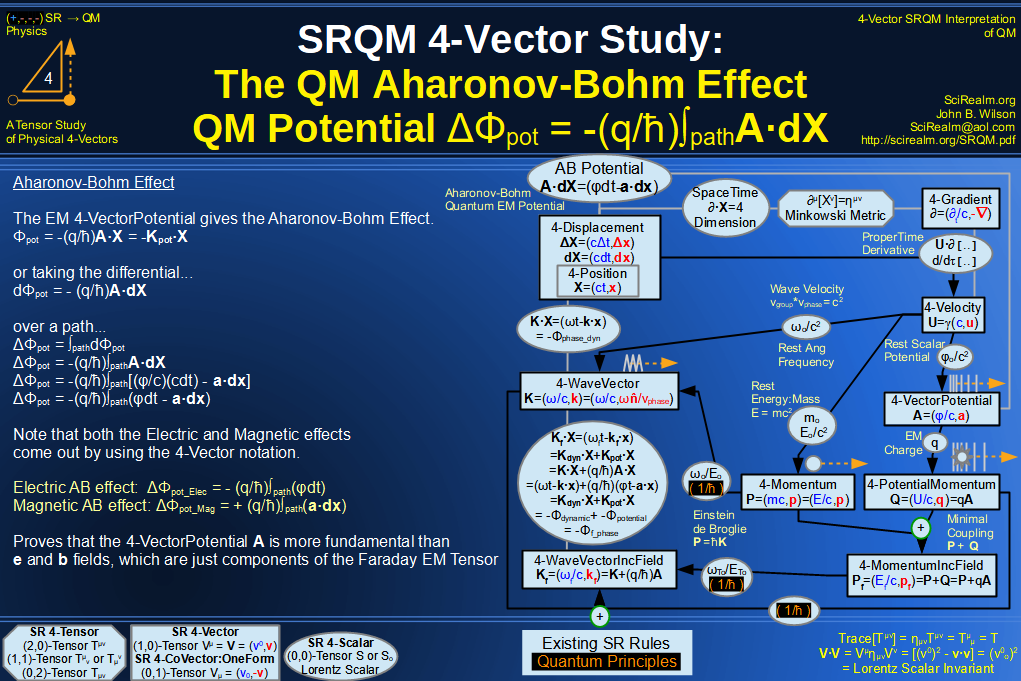SRQM 4-Vector : Four-Vector Josephson Junction Effect Diagram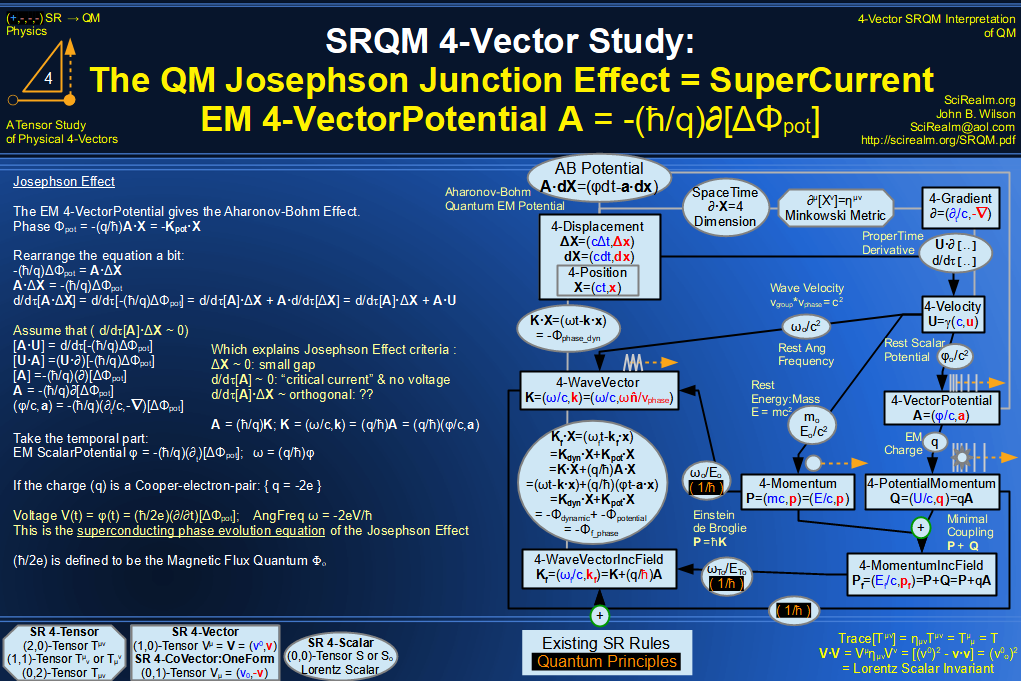SRQM 4-Vector : Four-Vector Hamilton-Jacobi vs Action, Josephson vs Aharonov-Bohm Diagram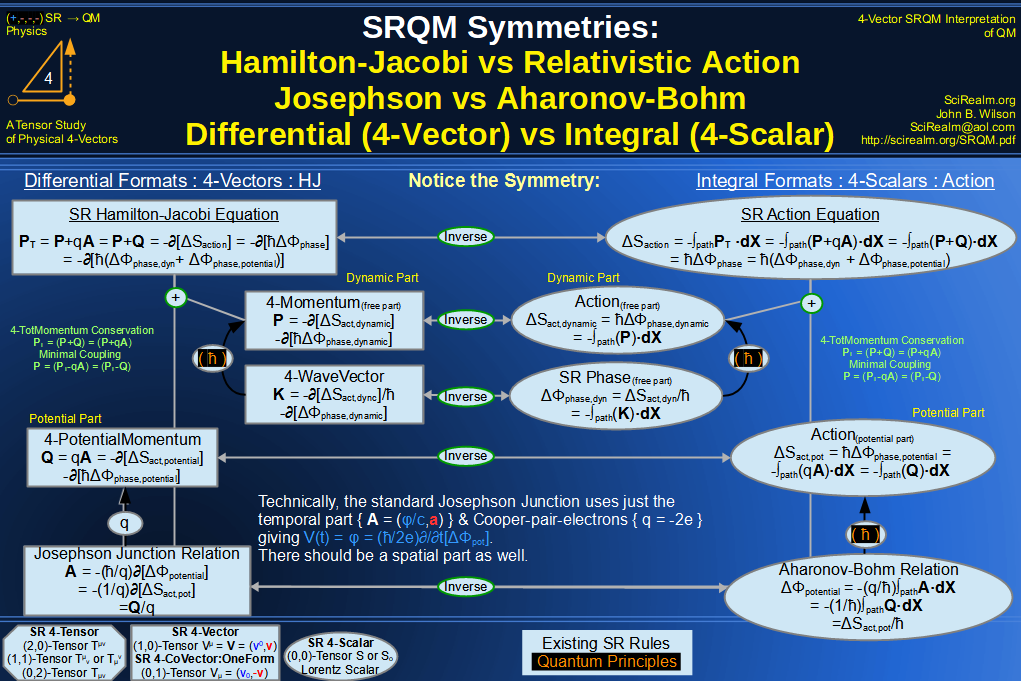SRQM 4-Vector : Four-Vector Motion of Lorentz Scalar Invariants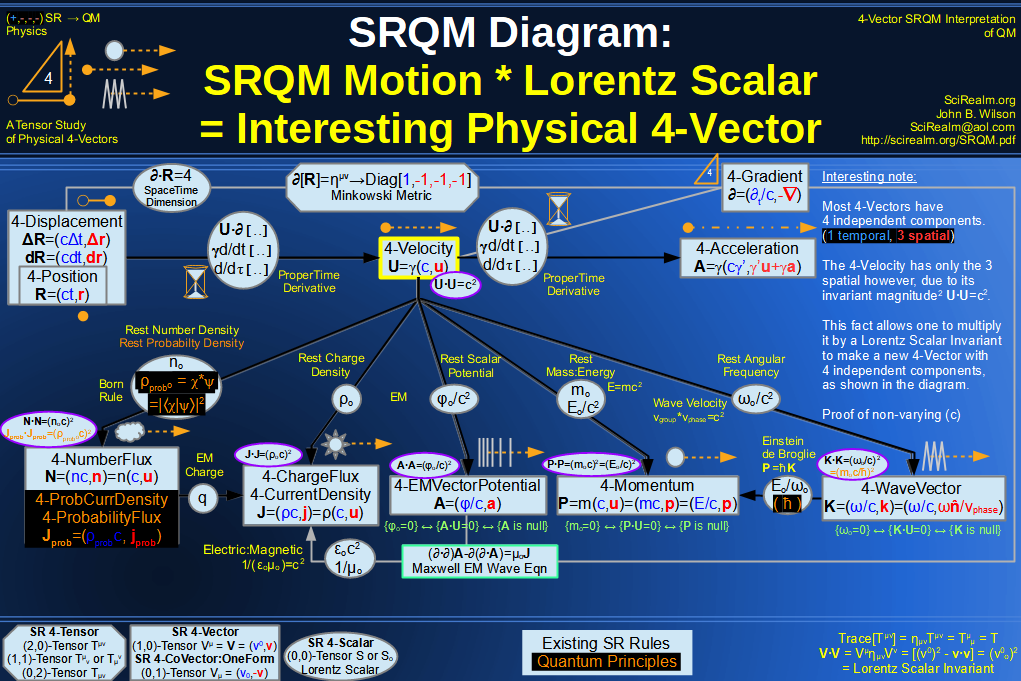SRQM 4-Vector : Four-Vector Motion of Lorentz Scalar Invariants, Conservation Laws & Continuity Equations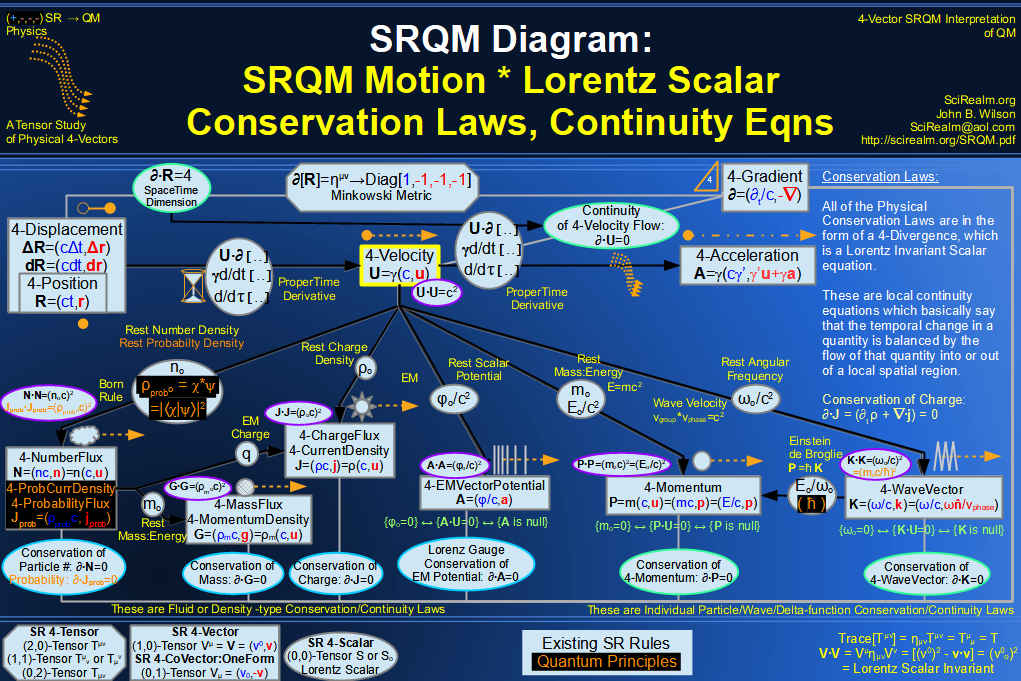SRQM 4-Vector : Four-Vector Speed of Light (c)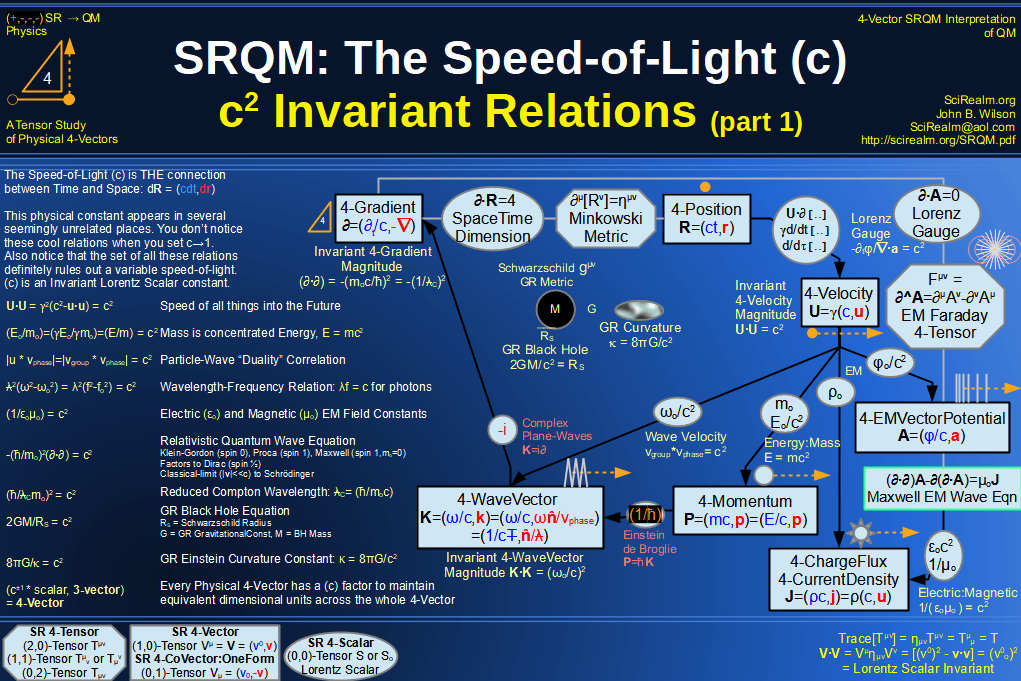SRQM 4-Vector : Four-Vector Minimal Coupling Conservation of 4-TotalMomentum)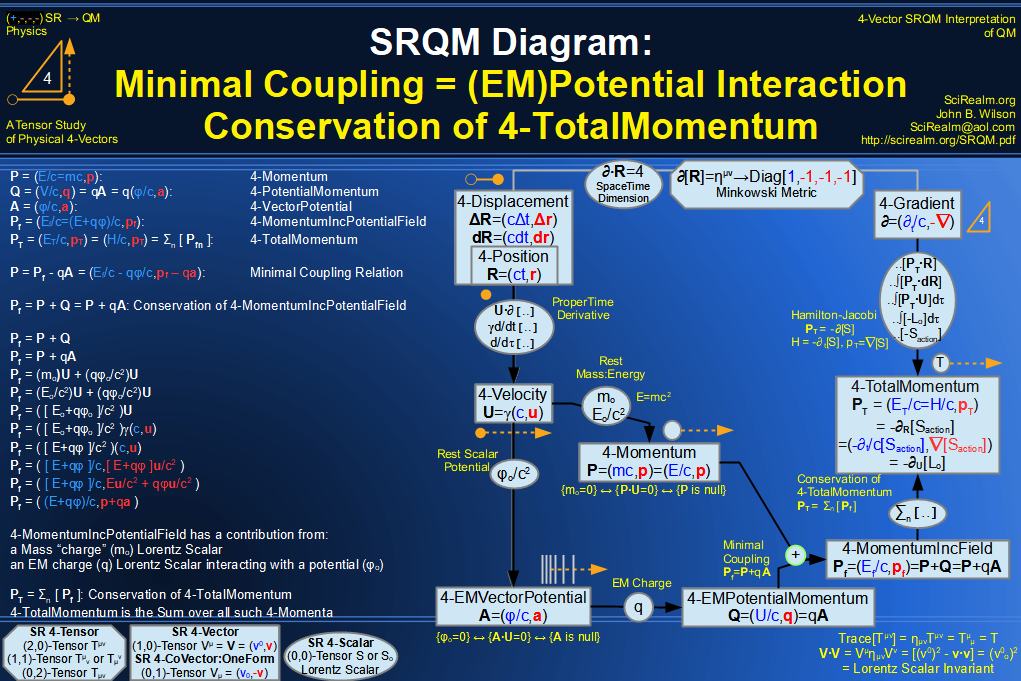SRQM 4-Vector : Four-Vector Relativistic Action (S) Diagram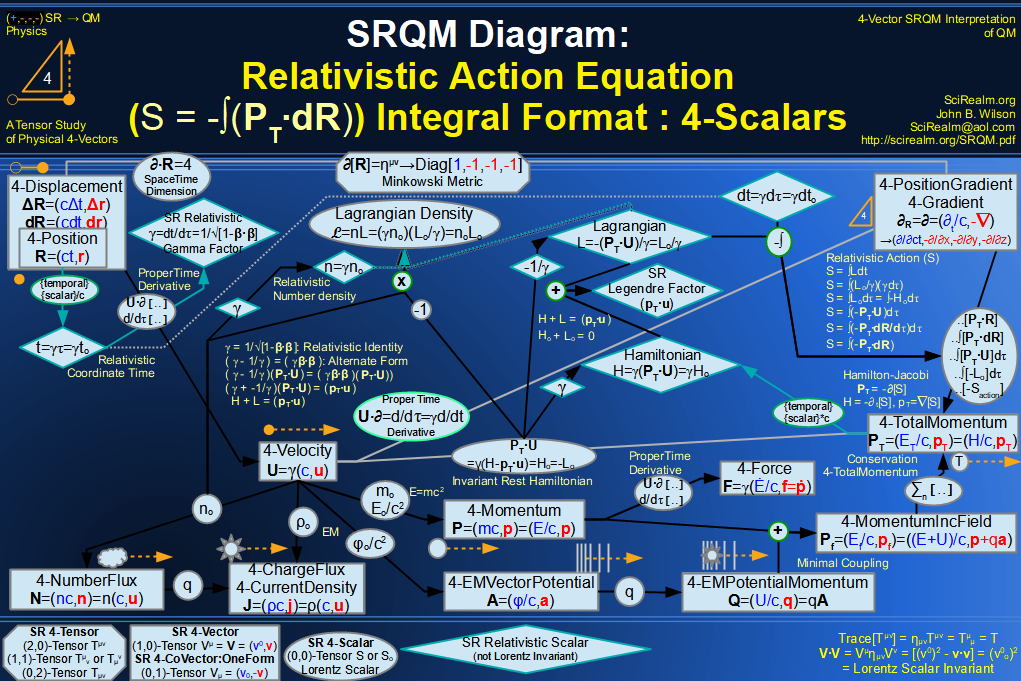SRQM 4-Vector : Four-Vector Relativistic Lagrangian Hamiltonian Diagram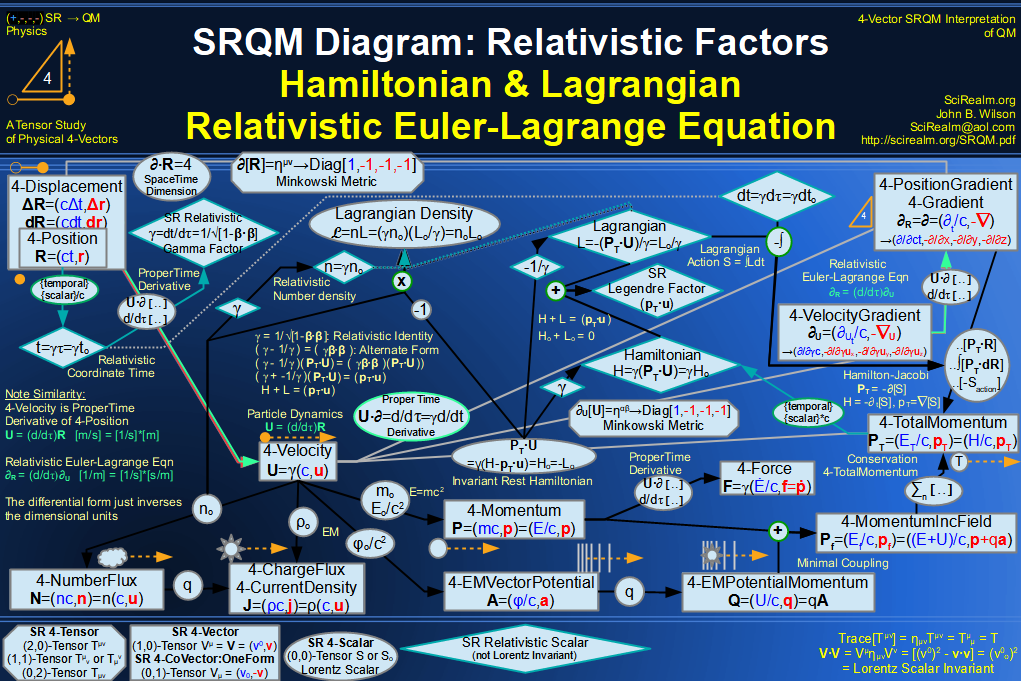SRQM 4-Vector : Four-Vector Relativistic Euler-Lagrange Equation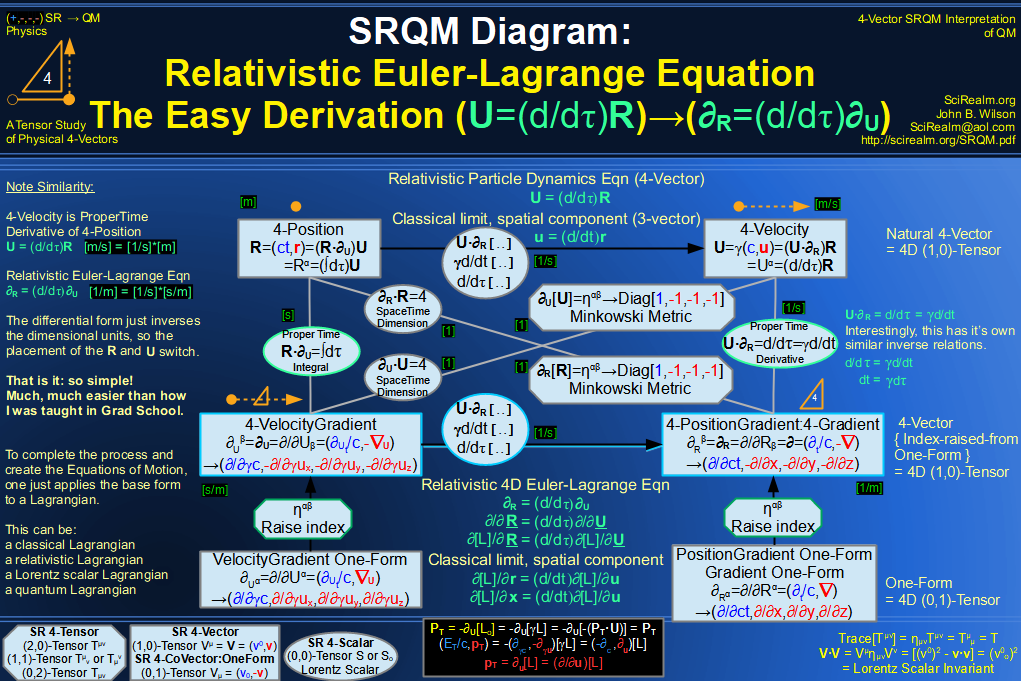SRQM 4-Vector : Four-Vector Relativistic EM Equations of Motion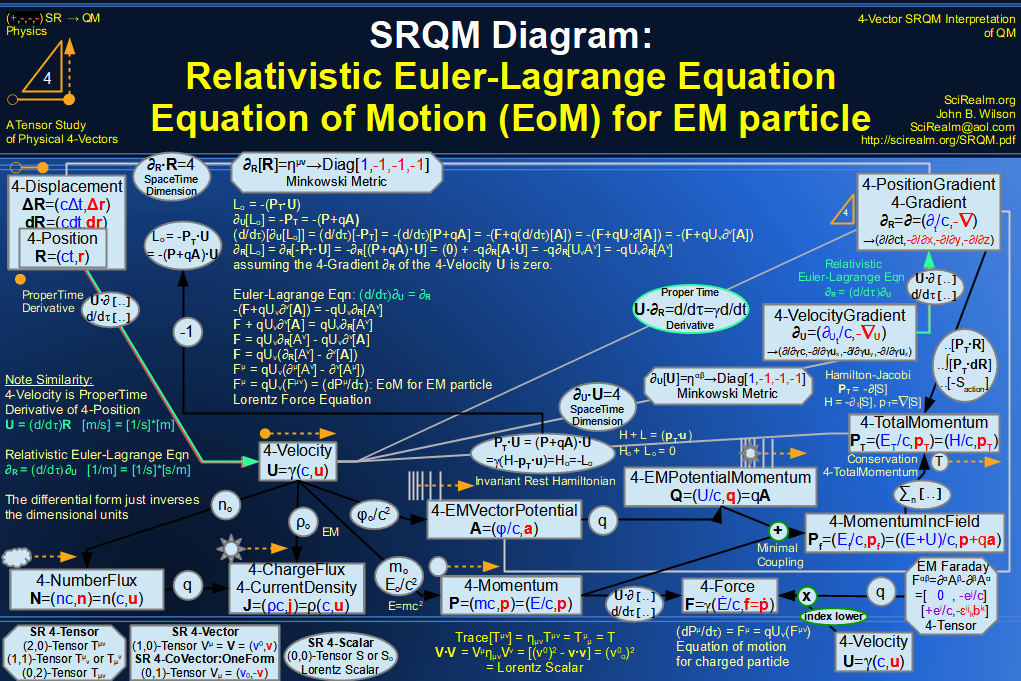SRQM 4-Vector : Four-Vector Einstein-de Broglie Relation hbar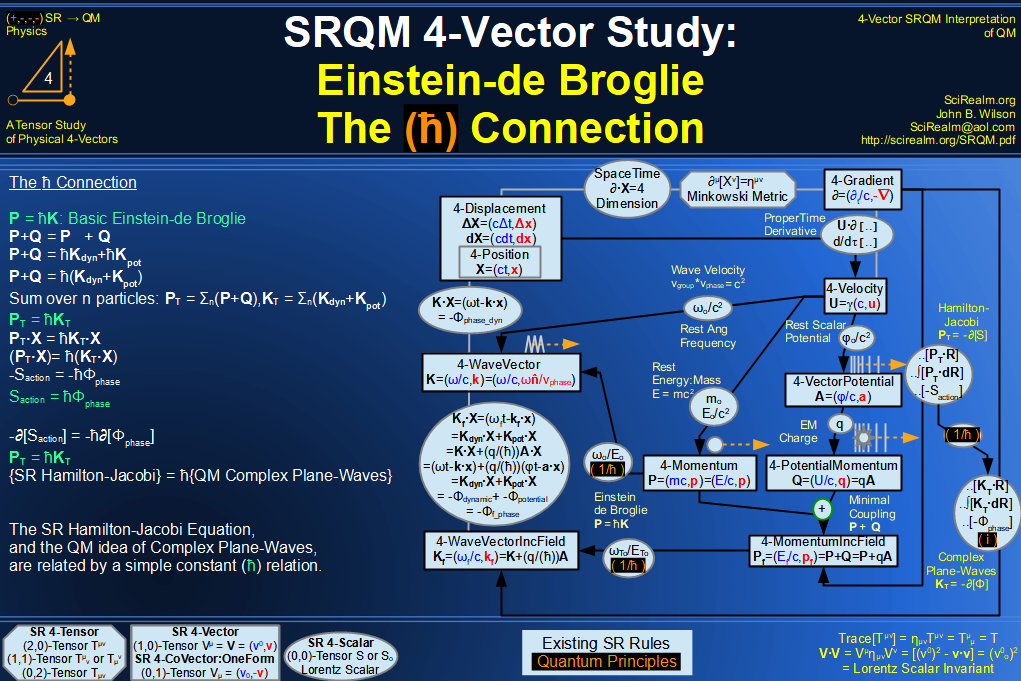SRQM 4-Vector : Four-Vector Quantum Canonical Commutation Relation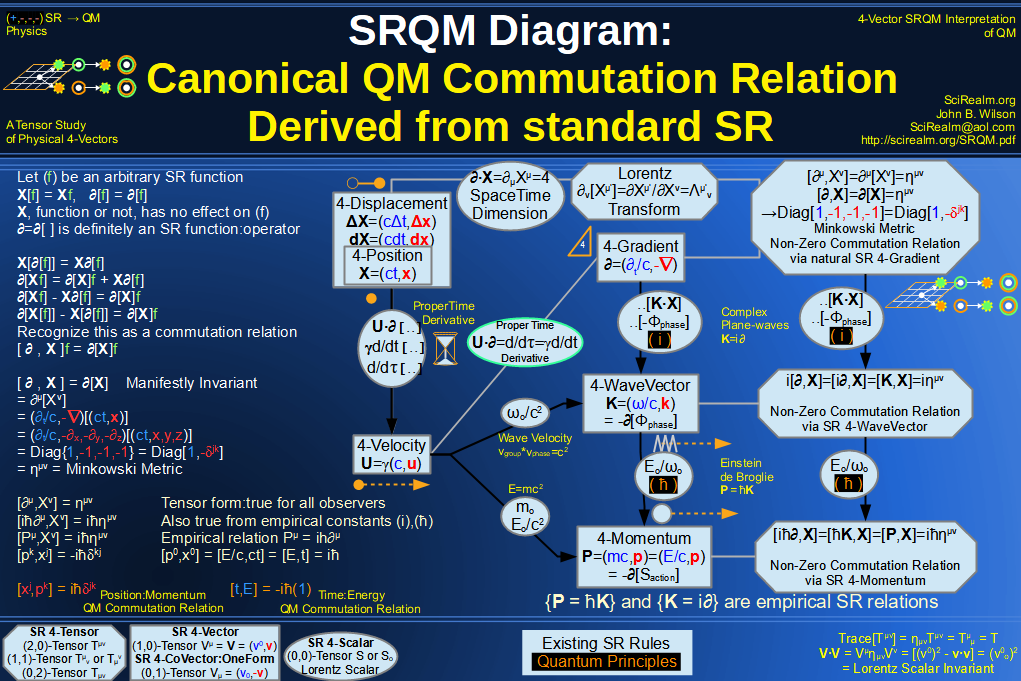SRQM 4-Vector : Four-Vector QM Schroedinger Relation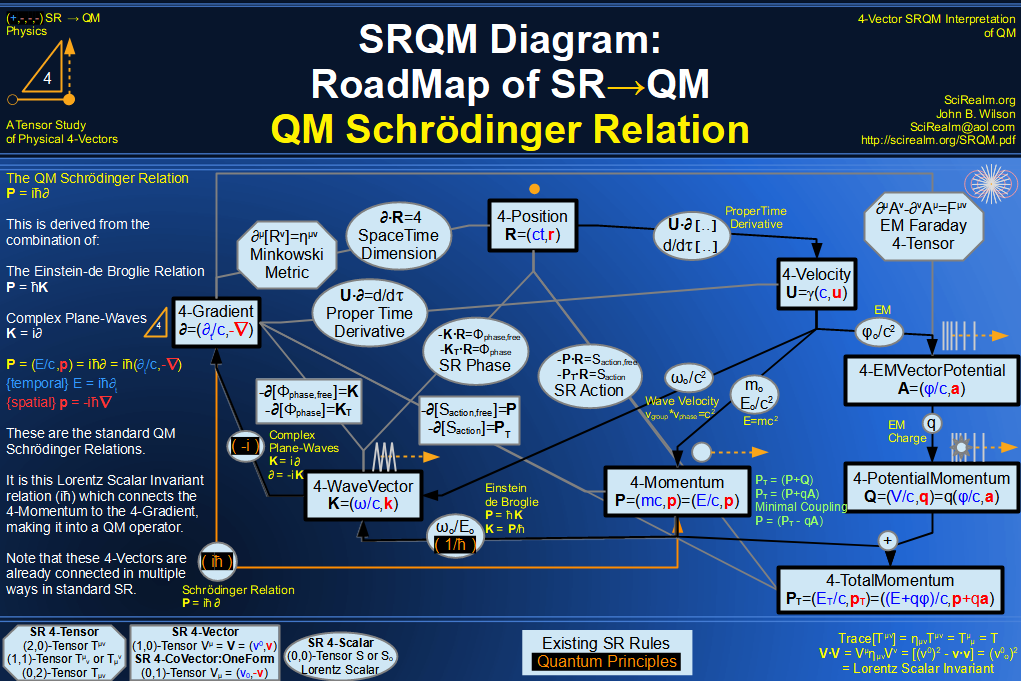SRQM 4-Vector : Four-Vector Quantum Probability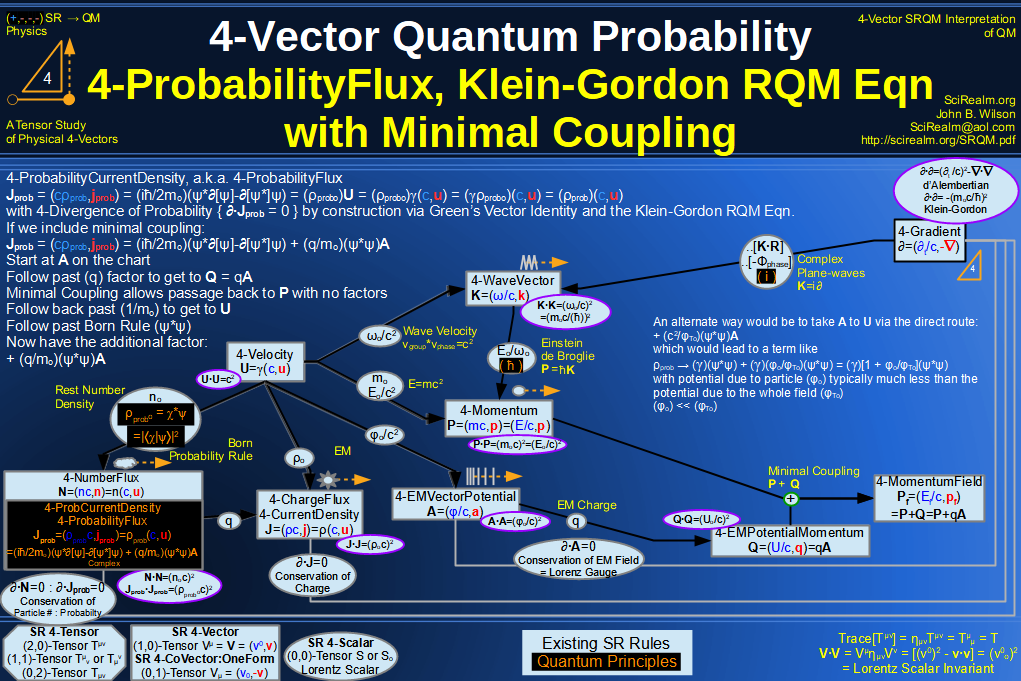SRQM 4-Vector : Four-Vector CPT Theorem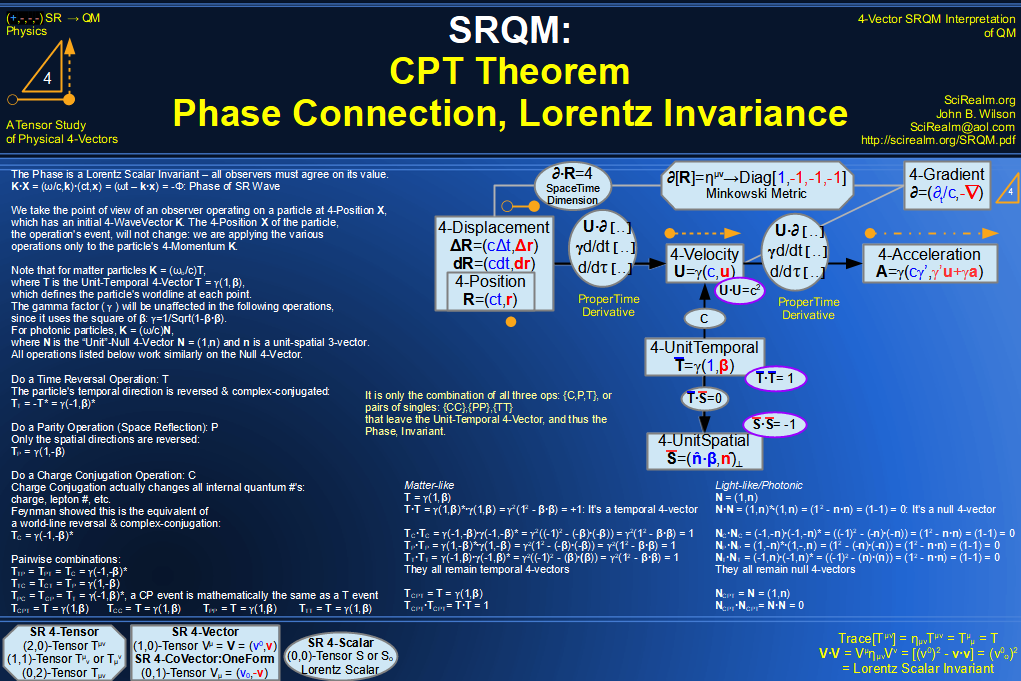SRQM 4-Vector : Four-Vector Lorentz Transforms Connection Map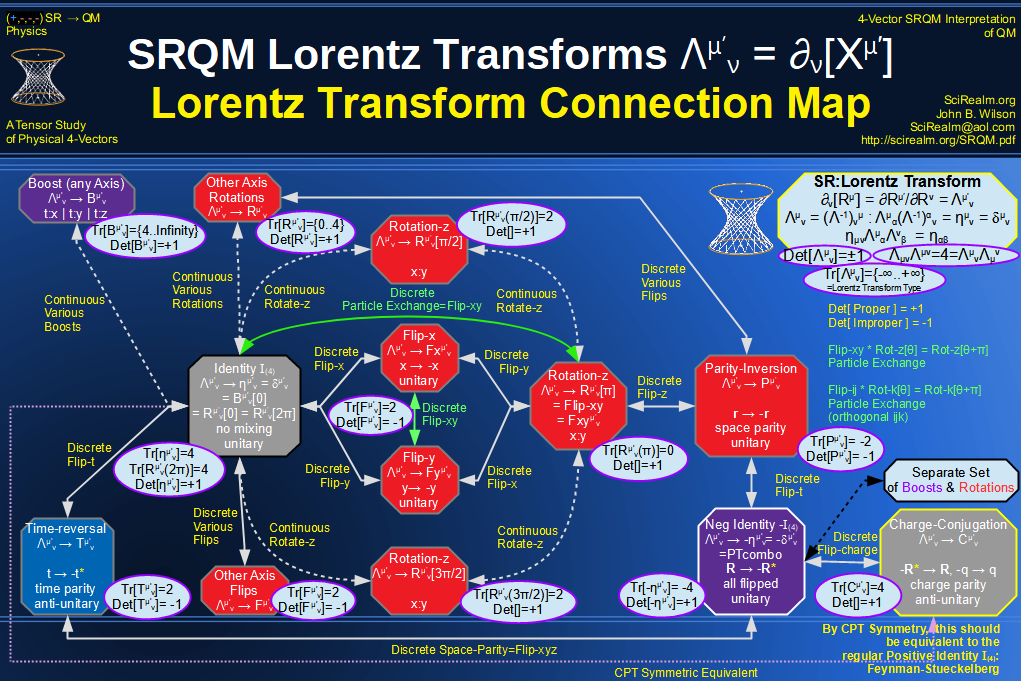SRQM 4-Vector : Four-Vector Lorentz Discrete Transforms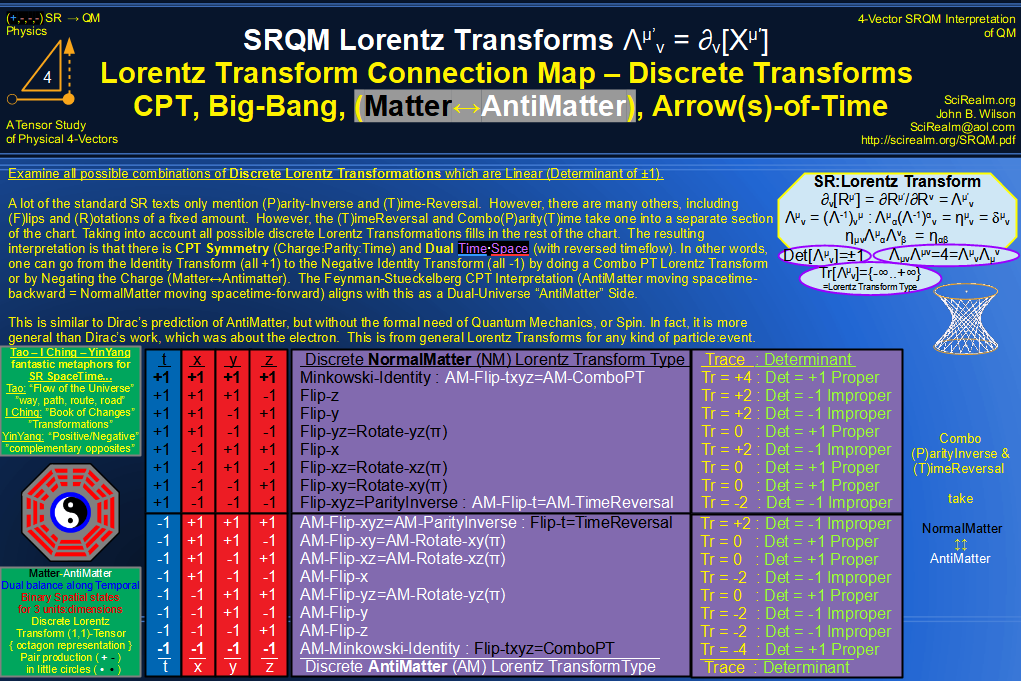SRQM 4-Vector : Four-Vector Lorentz Transforms - Trace Identification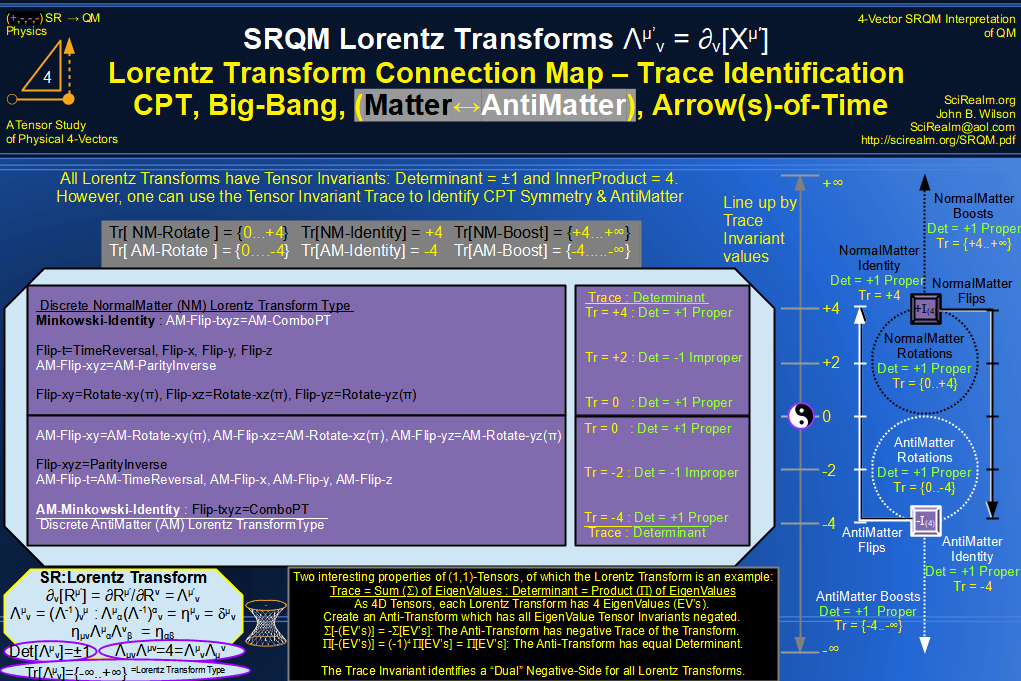SRQM 4-Vector : Four-Vector Lorentz Lorentz Transforms-Interpretations
CPT Symmetry, Baryon Asymmetry Problem Solution, Matter-Antimatter Symmetry Solution, Arrow-of-Time Problem Solution, Big-Bang!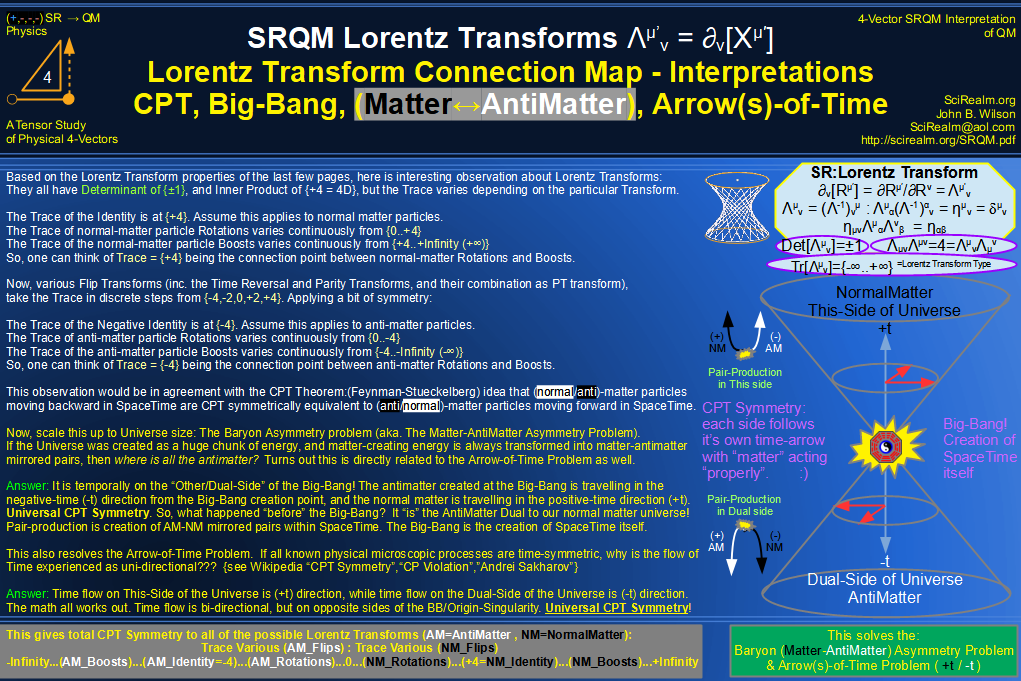See SRQM: QM from SR - The 4-Vector RoadMap (.html)
See SRQM: QM from SR - The 4-Vector RoadMap (.pdf)The Science Realm: John's Virtual Sci-Tech Universe John's Science & Math Stuff: | About John | Send email to John 4-Vectors | Ambigrams | Antipodes | Covert Ops Fragments | Cyrillic Projector | Forced Induction (Sums Of Powers Of Integers) | Fractals | Frontiers | JavaScript Graphics | Kid Science | Kryptos | Prime Sieve | QM from SR | Quantum Phase | Quotes | RuneQuest Cipher Challenge | Scientific Calculator | Secret Codes & Ciphers | Science+Math | Sci-Pan Pantheist Poems | Stereograms | Turkish Grammar |